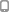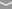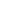•(219) 477-4555
•mjfrentals@yahoo.com

# Styled List Shortcode

Home / Styled List Shortcode

• type = arrow_down
• type = arrow_down
• type = arrow_down

• type = arrow_down_alt1
• type = arrow_down_alt1
• type = arrow_down_alt1

• type = arrow_left
• type = arrow_left
• type = arrow_left

• type = arrow_left_alt1
• type = arrow_left_alt1
• type = arrow_left_alt1

• type = arrow_right
• type = arrow_right
• type = arrow_right

• type = arrow_right_alt1
• type = arrow_right_alt1
• type = arrow_right_alt1

• type = arrow_up
• type = arrow_up
• type = arrow_up

• type = arrow_up_alt1
• type = arrow_up_alt1
• type = arrow_up_alt1

• type = bolt
• type = bolt
• type = bolt

• type = calendar_alt_fill
• type = calendar_alt_fill
• type = calendar_alt_fill

• type = chat
• type = chat
• type = chat

• type = chat_alt_fill
• type = chat_alt_fill
• type = chat_alt_fill

• type = check
• type = check
• type = check

• type = check_alt
• type = check_alt
• type = check_alt

• type = clock
• type = clock
• type = clock

• type = cog_alt
• type = cog_alt
• type = cog_alt

• type = comment_alt1
• type = comment_alt1
• type = comment_alt1

• type = denied
• type = denied
• type = denied

• type = home
• type = home
• type = home

• type = key_fill
• type = key_fill
• type = key_fill

• type = key_stroke
• type = key_stroke
• type = key_stroke

• type = lock_fill
• type = lock_fill
• type = lock_fill

• type = lock_stroke
• type = lock_stroke
• type = lock_stroke

• type = magnifying_glass
• type = magnifying_glass
• type = magnifying_glass

• type = mail
• type = mail
• type = mail

• type = mail_alt
• type = mail_alt
• type = mail_alt

• type = map_pin_fill
• type = map_pin_fill
• type = map_pin_fill

• type = map_pin_stroke
• type = map_pin_stroke
• type = map_pin_stroke

• type = minus_alt
• type = minus_alt
• type = minus_alt

• type = move_alt2
• type = move_alt2
• type = move_alt2

• type = movie
• type = movie
• type = movie

• type = new_window
• type = new_window
• type = new_window

• type = pen
• type = pen
• type = pen

• type = pen_alt_fill
• type = pen_alt_fill
• type = pen_alt_fill

• type = pin
• type = pin
• type = pin

• type = play
• type = play
• type = play

• type = play_alt
• type = play_alt
• type = play_alt

• type = plus
• type = plus
• type = plus

• type = plus_alt
• type = plus_alt
• type = plus_alt

• type = star
• type = star
• type = star

• type = tag_fill
• type = tag_fill
• type = tag_fill

• type = tag_stroke
• type = tag_stroke
• type = tag_stroke

• type = user
• type = user
• type = user

• type = x
• type = x
• type = x

```[list type="arrow_down"]
<ul>
<li>type = arrow_down</li>
</ul>
[/list]
```